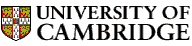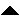Computer LaboratoryComputer Science Syllabus - Computer Systems ModellingComputer Systems ModellingNext: Denotational Semantics Up: Michaelmas Term 2005: Part Previous: Artificial Intelligence II   Contents

## Computer Systems Modelling

Lecturer: Dr R.J. Gibbens

No. of lectures: 12

Prerequisite course: Probability

Aims

The aims of this course are to introduce the concepts and principles of analytic modelling, operational analysis and simulation, with particular emphasis on understanding the behaviour of computer and communications systems.

Lectures

• Introduction to modelling. Overview of analytic techniques, operational analysis and simulation. Little's law.

• Introduction to discrete event simulation. Applicability to computer system modelling and other problems. Advantages and limitations of simulation approaches.

• Random number generation methods and simulation techniques. Review of statistical distributions. Statistical measures for simulations, confidence intervals and stopping criteria. Variance reduction techniques.

• Operational analysis. Simple operational quantities. Bottleneck analysis. Applicability and assumptions.

• Simple queueing theory. Stochastic processes: introduction and examples. The Poisson process. Markov chains. Advantages and limitations of analytic approaches.

• Birth-death processes, general flow balance equations. Relation to queueing systems. The M/M/1 queue in detail: solution for state occupancy, average queue length, average residence time. General observations.

• Queue classifications, variants on the M/M/1 queue and applications. Extensions to birth-death models. Queueing networks.

• The M/G/1 queue and its application. The Pollaczek-Khintchine formula; performance measures.

Objectives

At the end of the course students should

• be able to build simple Markov models and understand the critical modelling assumptions

• be able to solve simple birth-death processes

• understand that in general as the utilization of a system increases towards unity then the response time will tend to increase -- often dramatically so

• understand the tradeoffs between different types of modelling techniques

• be able to perform a bottleneck analysis of a system

• be aware of the issues in building a simulation of a computer system and analysing the results obtained

Reference books

* Ross, S.M. (2002). Probability models for computer science. Academic Press.
Jain, A.R. (1991). The art of computer systems performance analysis. Wiley.
Kleinrock, L. (1975). Queueing systems, vol. 1. Theory. Wiley.
Leung, C. (1988). Quantitative analysis of computer systems. Wiley.Next: Denotational Semantics Up: Michaelmas Term 2005: Part Previous: Artificial Intelligence II   Contents
Christine Northeast
Sun Sep 11 15:46:50 BST 2005© 2005 University of Cambridge Computer Laboratory Please send any comments to pagemaster@cl.cam.ac.uk Page last updated on 11-Sep-2005 at 15:57 by Christine Northeast